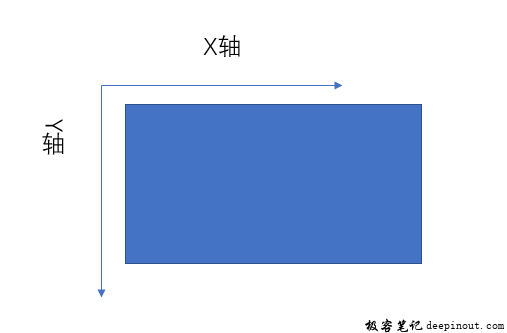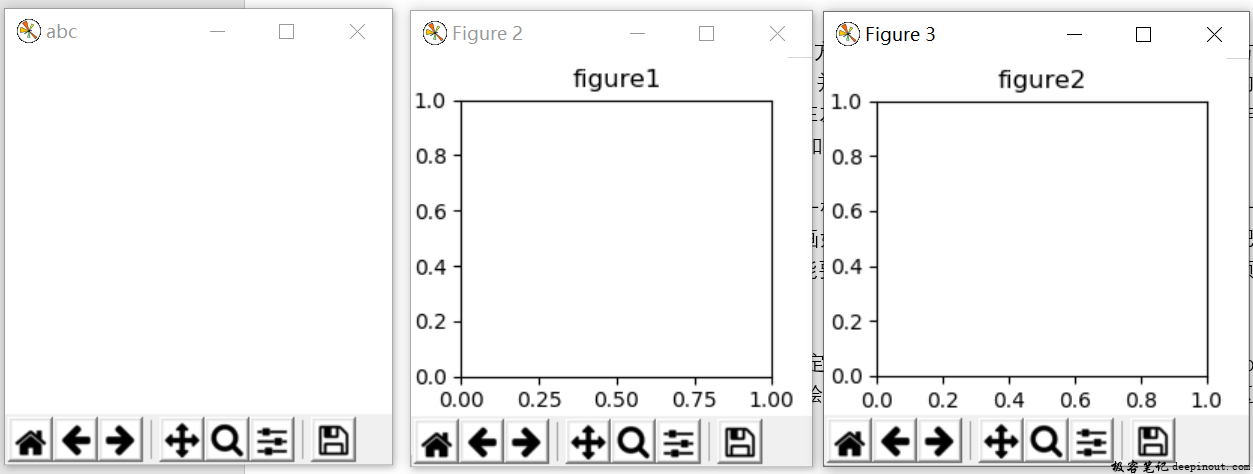# Matplotlib 绘图区域import matplotlib.pyplot as plt
import numpy as np

fig = plt.figure('abc')
f1 = plt.figure()
plt.title("figure1")
f2 = plt.figure()
plt.title("figure2")
plt.show()


• 回顶